# Non-interference analysis of delegation subterfuge in distributed authorization systems

## Abstract

A principal carrying out a delegation may not be certain about the state of its delegation graph as it may have been perturbed by an attacker. This perturbation may come about from the attacker concealing the existence of selected delegation certificates and/or injecting new delegation certificates. As a consequence of this delegation subterfuge the principal may violate its own policy that guides delegation actions. This paper considers the verification of the absence of subterfuge in systems that accept and issue delegation certificates. It is argued that this absence of subterfuge is not a safety property and a non-interference style security-property based interpretation is proposed.

## Introduction

Trust Management systems – provide a decentralized approach for managing delegation of trust between principals. These systems are typically explicit in their assumption that principals can be tied to an unambiguous identification, for example, Alice with her unique public key. However, the literature has generally not been as prescriptive in terms of how permission identifiers should be tied to the actions that they authorize. While central authorities such as the Internet Corporation for Assigned Names and Numbers (ICANN) might, in principle, provide identifiers that could be used for this purpose, a malicious principal can still choose to ignore or misrepresent the interpretation. A delegation subterfuge attack , can occur when there is the potential for ambiguity in interpreting a delegated permission. This can come about from an attacker perturbing a victim’s delegation graph by concealing and/or injecting delegation certificates. As a consequence, the victim may violate the requirements that guide its own delegation actions. It is argued  that the problem of delegation subterfuge is analogous to the problem of a message freshness-attack.

A number of delegation subterfuge scenarios and defense mechanisms have been previously considered ,,. However, while demonstrating its existence, this previous research did not provide a formal definition of subterfuge and, instead, relied on ad-hoc delegation mechanisms that, intuitively, defend against the attack. Rather than presuming correct operation of these ad-hoc mechanisms, in this paper we are interested in characterizing what is meant by subterfuge and in designing delegation mechanisms that can be proven to be subterfuge-free.

In this paper we consider the verification of the absence of subterfuge in applications and mechanisms that accept and issue delegation certificates. Using a running example, we argue that subterfuge-freedom should not be treated as an Alpern-Schneider  safety-style property. It is insufficient for an application to decide whether it is safe to delegate, based on its view of a delegation graph (current state), as this view may have been perturbed by an attacker. In deciding whether to delegate, the application must also consider that other delegation graph configurations exist that may be as equally valid as the current state, based on the available information. Therefore, we conjecture that subterfuge-freedom is not a property on a single state but a property on sets of states. A non-interference , style property is proposed for freedom from delegation subterfuge. Demonstrating and encoding subterfuge-freedom as a security (non-interference) property is the primary contribution of this paper.

The paper is organized as follows. In Section ‘Authorization delegation’ we describe a simple SPKI-style delegation model that is sufficient to present the results of this paper. Section ‘Delegation subterfuge’ presents an example of a subterfuge attack on a service reseller application that uses delegation to manage trust relationships. Section ‘Delegation as a safety property’ argues that treating the problem as a safety property is insufficient as it does not prevent the subterfuge-attack in the application. Section ‘Delegation as a security property’ proposes a non-interference style property to characterize subterfuge freedom and demonstrates its interpretation in the service reseller example. Related work is discussed in Section ‘Related work’ and Section ‘Discussion and conclusion’ concludes the paper.

## Authorization delegation

Principals are active entities that can source and sink messages. We assume that a principal P who owns a public key K P knows its corresponding private key ${K}_{P}^{-1}$ and can use this private key to sign a message M, denoted as ${\left\{\phantom{\rule{0.3em}{0ex}}|\phantom{\rule{0.3em}{0ex}}M\phantom{\rule{0.3em}{0ex}}|\phantom{\rule{0.3em}{0ex}}\right\}}_{s{K}_{P}}$. By validating the signature, it is easy for a third party to confirm that the signed message ${\left\{\phantom{\rule{0.3em}{0ex}}|\phantom{\rule{0.3em}{0ex}}M\phantom{\rule{0.3em}{0ex}}|\phantom{\rule{0.3em}{0ex}}\right\}}_{s{K}_{P}}$ originated from the owner of K P . In this paper, when no ambiguity can arise, we interchangeably refer to a principal by its name P or by the public key K P that is owned by P.

A delegation certificate is a statement that has been signed by a principal who owns public key K P stating that that it trusts another principal Q for some permission X. This trust may be conditional, for example, a SPKI delegation certificate ${\left\{\phantom{\rule{0.3em}{0ex}}|\phantom{\rule{0.3em}{0ex}}Q,X,D,V\phantom{\rule{0.3em}{0ex}}|\phantom{\rule{0.3em}{0ex}}\right\}}_{s{K}_{P}}$ specifies a validity period V on the delegation, and a delegation bit D indicates whether Q may further delegate this permission X. Note that in this paper we do not consider the delegation validity period and assume that every delegated permission may be further delegated (delegation bit D=1). This assumption, however, does mean that we limit ourselves to transitive delegation (reflected by Rule D3 below). However, the results in this paper can, in principle, be generalized to other delegation models that support intransitive delegation; our simplification is made for the sake of ease of exposition.

A simple model is developed that describes how collections of delegation certificates may be interpreted.

Delegation statement. A delegation statement $P\stackrel{X}{\to }Q$ specifies that principal P delegates authority for permission X to principal Q.

Certificates. A delegation certificate is a signed message ${\left\{\phantom{\rule{0.3em}{0ex}}|\phantom{\rule{0.3em}{0ex}}Q,X\phantom{\rule{0.3em}{0ex}}|\phantom{\rule{0.3em}{0ex}}\right\}}_{s{K}_{P}}$, whereby principal owning key K P states that it trusts principal Q for permissions X. The following inference rule (identified as Rule D 1) provides an interpretation for this certificate as a statement:

$\frac{{\left\{\phantom{\rule{0.3em}{0ex}}|\phantom{\rule{0.3em}{0ex}}Q,X\phantom{\rule{0.3em}{0ex}}|\phantom{\rule{0.3em}{0ex}}\right\}}_{s{K}_{P}}}{{K}_{P}\stackrel{X}{\to }Q}\phantom{\rule{1em}{0ex}}\left[\phantom{\rule{0.3em}{0ex}}D1\right]$

This is specified as a basic rewrite rule: given ${\left\{\phantom{\rule{0.3em}{0ex}}|\phantom{\rule{0.3em}{0ex}}Q,X\phantom{\rule{0.3em}{0ex}}|\phantom{\rule{0.3em}{0ex}}\right\}}_{s{K}_{P}}$, we can infer ${K}_{P}\stackrel{X}{\to }Q$.

Permissions. We assume that there exists a set of permissions PERM that forms a preorder under relation $⊑$. $X⊑Y$ denotes permission ordering, whereby $X⊑Y$ is interpreted to mean that permission X provides no less authorization than Y and XY defines permission intersection. Intuitively, a principal authorized for permission Y is also authorized for any permission X where $X⊑Y$. Thus, we have, for any principals P,Q and permission X then

$\frac{P\stackrel{Y}{\to }Q;\phantom{\rule{0.3em}{0ex}}X⊑Y}{\phantom{\rule{-33.0pt}{0ex}}P\stackrel{X}{\to }Q}\phantom{\rule{1em}{0ex}}\left[\phantom{\rule{0.3em}{0ex}}D2\right]$

Intuitively, this interpretation of permissions follows that used by SPKI/KeyNote. For example, in SPKI the set of all possible s-expression permission tags (PERM) define a preorder, with tag intersection providing a greatest lower bound operation. Thus, for instance, the relationship

$\mathsf{\text{(tag (http alice.com/view?p))}}⊑\mathsf{\text{(tag (http (* prefix alice.com/)))}}$

holds between the given s-expression permission tags. Conventional Trust Management systems, such as SPKI and KeyNote, implicitly assume that the preorder that is used to compare permissions is centralized, that is, it is a priori defined and globally known to all principals. Trust Management systems that support a decentralized preorder that is defined and extended on the fly by the principals is non-trivial and leads to challenges , that are not considered by the model in this paper.

Delegation reduction. Collections of delegation statements may be reasoned over using SPKI  style certificate reduction. Given principals P,Q,R and permissions X and Y then we define:

$\frac{P\stackrel{X}{\to }Q;\phantom{\rule{0.3em}{0ex}}Q\stackrel{Y}{\to }R;}{\phantom{\rule{-44.0pt}{0ex}}P\stackrel{X\sqcap Y}{\to }R}\phantom{\rule{1em}{0ex}}\left[\phantom{\rule{0.3em}{0ex}}D3\right]$

This rule reflects the assumption in this paper that delegation is transitive.

Given a collection of delegation statements/certificates, then the model described in this section can be used to answer questions such as “does P trusts Q for permission X?”, that is, is it possible to infer the statement $P\stackrel{X}{\to }Q$ using inference Rules D 1– D 3.

There are features of Trust Management/authorization models described in the literature that are not considered in this paper. The model used in this paper is closest to SPKI  and KeyNote , since their interpretation of (transitive) delegation follows Rules D 1– D 3. Other Trust Management models may support more expressive and complex delegation statements. In selecting our relatively simple model of delegation, our intention is to elucidate the concept of subterfuge in a manner that is not encumbered by the details that a more sophisticated model might offer.

## Delegation subterfuge

Trust Management systems are typically explicit in their assumption that principals are uniquely identified, for example, using a public key to reliably identify a principal. However, the literature has generally not been as prescriptive regarding the uniqueness of permissions. The threat of delegation subterfuge arises when there is ambiguity concerning the uniqueness and interpretation of a permission. This is illustrated by the following running example.

### Example: trust management for service reselling

Principal Reese agrees to act as a reseller of hotel rooms offered by Harry and Mike. When reselling a room, Reese decides a room resell rate that is based on her business contract with the hotel and issues customers with an unforgable room resell rate agreement to be presented on arrival to the hotel. Customers pay the hotel directly for their room according to the amount specified in the resell rate. The arrangement is that, in reselling a room, Reese provides the hotel with a guaranteed room rate. Any surplus between the room resell rate and the guaranteed rate is passed on to Reese, while Reese is liable for any deficit.

The trust relationships in this example are encoded as delegation statements using the model presented in the previous section. Any reasoning about delegation in this example is done according to Rules D 1– D 3.

Reseller Reese and hotel Harry enter contracts by issuing delegation statements

$\mathit{\text{Reese}}\stackrel{\mathsf{\text{contract}}\left(r,v\right)}{\to }\mathit{\text{Harry}};\phantom{\rule{1em}{0ex}}\phantom{\rule{1em}{0ex}}\phantom{\rule{1em}{0ex}}\phantom{\rule{1em}{0ex}}\mathit{\text{Harry}}\stackrel{\mathsf{\text{contract}}\left(r,v\right)}{\to }\mathit{\text{Reese}}$

for guaranteed rate v for room r. Harry subsequently issues

$\mathit{\text{Harry}}\stackrel{\mathsf{\text{resell}}\mathrm{.r.}\ast }{\to }\mathit{\text{Reese}}$

delegating reselling authority for room r to Reese. In this example the second attribute of permission resell specifies the rate at which the room is sold and the wildcard “ ” reflects that Harry places no constraint on the resell rate. We can treat the wildcard as an upper bound on resell permissions whereby $\mathsf{\text{resell}}\mathrm{.r.u}⊑\mathsf{\text{resell}}\mathrm{.r.}\ast$, that is, a holder of permission resell.r.* is authorized (by inference rule D 2) to resell the room for any rate u. In general, a principal authorized to resell a room at rate v can also sell it at any rate u higher than v, that is, $\mathsf{\text{resell}}\mathrm{.r.u}⊑\mathsf{\text{resell}}\mathrm{.r.v}\phantom{\rule{2.77626pt}{0ex}}⇔\phantom{\rule{2.77626pt}{0ex}}v\le u$. Thus, if Reese guarantees a \$50 room rate for Harry then she can sell the room at any higher rate (to her benefit). For example, on reselling this room to Clare for \$60, Reese issues

$\mathit{\text{Reese}}\stackrel{\mathsf{\text{resell}}\mathrm{.r.}60}{\to }\mathit{\text{Clare}}$

On check-in, Clare presents delegation chain

$\mathit{\text{Harry}}\stackrel{\mathsf{\text{resell}}\mathrm{.r.}\ast }{\to }\mathit{\text{Reese}};\mathit{\text{Reese}}\stackrel{\mathsf{\text{resell}}\mathrm{.r.}60}{\to }\mathit{\text{Clare}}$

which Harry reduces (by Rule D 3) to $\mathit{\text{Harry}}\stackrel{\mathsf{\text{resell}}\mathrm{.r.}60}{\to }\mathit{\text{Clare}}$, as proof that Clare is authorized for the \$60 room rate. This chain, along with the contract certificates are used by Harry and Reese in claiming reimbursement of any deficit/surplus (in this case, a surplus for Reese).

We are not concerned with the claim process in this paper, however, we are interested in Reese ensuring that she never resells a room below some minimum rate minRate that she decides. Table 1 gives sample guaranteed (contracted) and minimum rates for any rooms in hotels Harry and Mike. We assume that Reese may be willing to sell a room at a loss, for example, when it is bundled as part of a package that is profitable overall.

These minimum rates are decided by Reese, and are represented as a delegation statement $\mathit{\text{Reese}}\stackrel{\mathsf{\text{rate}}\mathrm{.v}}{\to }\mathit{\text{Harry}}$ indicating that Reese is willing to resell any room in hotel Harry at rate v or higher. For simplicity we assume that all rooms in the hotel are the same. If Reese is willing to resell a room at rate v then it follows she is willing to resell the room at any higher rate u where uv; thus, we define the permission ordering $\mathsf{\text{rate}}\mathrm{.u}⊑\mathsf{\text{rate}}\mathrm{.v}\phantom{\rule{2.77626pt}{0ex}}⇔\phantom{\rule{2.77626pt}{0ex}}u\ge v$. Note that this rate delegation statement is used by Reese internally to implement the minRate relationship and it is not necessary for her to share the corresponding certificates with any other principal. For example, $\mathit{\text{Reese}}\stackrel{\mathsf{\text{rate}}.40}{\to }\mathit{\text{Harry}};\mathit{\text{Reese}}\stackrel{\mathsf{\text{rate}}.10}{\to }\mathit{\text{Mike}}$ implement the minimum rates in Table 1.

The service reselling example illustrates how trust relationships between principals can be encoded as delegation statements and used by an application to decide whether it is safe to carry out a (check-in) operation.

### Subterfuge

Consider the following reselling scenario. Suppose that (malicious) Mike interferes with communication between hotel Harry and reseller Reese, intercepts the delegation certificate $\mathit{\text{Harry}}\stackrel{\mathsf{\text{resell.r.*}}}{\to }\mathit{\text{Reese}}$, and replaces it by $\mathit{\text{Mike}}\stackrel{\mathsf{\text{resell.r.*}}}{\to }\mathit{\text{Reese}}$, leading Reese to believe that permission resell.r.* is related to a room at Mike’s hotel. Eve, who is colluding with Mike, then uses Reese’s website to book this room r for a cost of \$20, in compliance with Reese’s minimum-rate policy in Table 1. Reese issues a certificate for $\mathit{\text{Reese}}\stackrel{\mathsf{\text{resell.r.20}}}{\to }\mathit{\text{Eve}}$. However, Eve obtains the intercepted certificate $\mathit{\text{Harry}}\stackrel{\mathrm{resell.r.}\ast }{\to }\mathit{\text{Reese}}$ from Mike and offers this, along with $\mathit{\text{Reese}}\stackrel{\mathsf{\text{resell.r.20}}}{\to }\mathit{\text{Eve}}$, as proof to Harry that she is authorized for this rate at his hotel.

There are other variations of subterfuge . For example, Reese has a legitimate expectation that so long as she delegates competently then she should not be liable for any confusion that is a result of poor permission design. Perhaps Harry and Reese collude in order to provide plausible deniability on a resold room. On believing she (Clare) paid Reese for an expensive room in Harry’s hotel ($\mathit{\text{Reese}}\stackrel{\mathsf{\text{resell.r.100}}}{\to }\mathit{\text{Clare}}$), Harry presents $\mathit{\text{Mike}}\stackrel{\mathsf{\text{resell.r.*}}}{\to }\mathit{\text{Reese}}$ to Clare arguing that she actually bought a room at Mike’s (cheap) hotel. In this case the existence of a delegation chain ($\mathit{\text{Harry}}\stackrel{\mathsf{\text{resell.r.*}}}{\to }\mathit{\text{Reese}},\mathit{\text{Reese}}\stackrel{\mathsf{\text{resell.r.100}}}{\to }\mathit{\text{Clare}}$) is insufficient to provide adequate accountability on Reese for the rooms she resells. These examples of subterfuge can be thought of as a variation on a semantic attack  at the interface between systems rather than necessarily between humans and computers. In this case the attacker Mike targets the way that Reese (a system) assigns meaning to content (a permission).

Certificate chains have been used in the literature to support degrees of accountability of authorisation –. The micro-billing scheme  uses KeyNote to help determine whether a micro-check (a KeyNote credential, signed by a customer) should be trusted and accepted as payment by a merchant. In , delegation credentials are used to manage the transfer of micropayment contracts between public keys; delegation chains provide evidence of contract transfer and ensure accountability for double-spending. The mechanisms that underly these schemes are similar to our service-reselling example in that there can be misinterpretation as to which principal (bank) originates the permission (authority for payment). These systems are also vulnerable to delegation subterfuge (leading to a breakdown in accountability) if care is not taken to properly identify the permissions indicating the payment authorizations when multiple banks and/or provisioning agents are possible.

It could be argued that the inadequacy in the permission design in our example is ‘obvious’ and that additional information should be included in the name of the permission. For example, one could argue that permission mike.com/resell.r.20 is clearly related to Mike’s website/hotel. However, on receipt of a certificate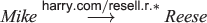Reese may unwittingly delegate $\mathit{\text{Reese}}\stackrel{\mathsf{\text{harry.com/resell.r.20}}}{\to }\mathit{\text{Eve}}$, not understanding that Mike has no authority over harry.com and that the intercepted certificate $\mathit{\text{Harry}}\stackrel{\mathsf{\text{harry.com/resell.r.20}}}{\to }\mathit{\text{Reese}}$ can be used by Eve to obtain a room at Harry’s hotel for \$20. Furthermore, design of the permission harry.com/resell.r.* assumes that there is a non-transient association between the domain harry.com and a (hotel) principal. However, domain name owners change in practice, intentionally or otherwise , and therefore, permission harry.com/resell.r.* should not be considered to necessarily specify an unambiguous authorization.

Arguing that prior to issuing a delegation statement that Reese has a responsibility to confirm that Mike owns the Harry.com domain is unsatisfying. This presumes separate and properly operating processes of managing a public key infrastructure, authenticating Mike’s identity and checking it against the permission. Furthermore, it places part of the reasoning about authorization outside of the rules D 1– D 3 of our authorization model, which is contrary to the intent of a Trust Management system . Moreover, with the declining numbers of system administrators relative to the number of Internet domains , we envisage that it becomes more difficult for organizations to maintain a consistent view of the relationships between domains and permissions.

### Eliminating subterfuge

Various ad-hoc modifications to our delegation model can be made to ensure unambiguous interpretation of a permission. For example, on the basis that public keys are considered unique and if Harry owns public key K H then signed permission ${\left\{\phantom{\rule{0.3em}{0ex}}|\phantom{\rule{0.3em}{0ex}}\mathsf{\text{resell.r.*}}\phantom{\rule{0.3em}{0ex}}|\phantom{\rule{0.3em}{0ex}}\right\}}_{s{K}_{H}}$ provides a unique and unambiguous permission identifier that can be tied to Harry. However, in order for this scheme to avoid subterfuge, the recognition of a permission string such asis required, which is, in itself, subject to confusion by a principal.

Instead of using public keys, one might be tempted to use SDSI-like local names  to make this task more manageable for Bob. However, in order to prevent subterfuge, permissions require a name that is unique across all name spaces where it will be used, not just the local name space of Bob. In Bob’s local name space the permission 〈(Bob’s Harry):resell.r.*〉 might refer to a different Harry to the Harry that Reese knows. Ensuring consistent interpretation among these locally named permissions is a non-trivial task .

Another possible source of suitable permission identifiers is a global X500-style naming service (if it could be built) that would tie global identities to real world entities, that would in turn be used within permissions. X500 Distinguished Names are, by definition, globally unique. If it were referenced in an extended validation certificate  then it is, in some legal sense, unambiguous, and is therefore not subject to subterfuge. However, X500-style approaches suffer from a variety of practical problems  when used to keep track of the identities of principals. In the context of subterfuge, a principal might easily be confused between the (non-unique) common name and the global distinguished name contained within a permission that used such identifiers.

One practical difficulty when relying on public keys as global identifiers is that their use is often transitory. A public key serves as an identifier (for its owner) for as long as the key is regarded as valid. If the (private) key is compromised, or if the owner decides to re-key then authorization certificates will have to be re-issued by all participants on delegation chains involving the permission. If K M re-keys to K M′, and issues a new certificate ${\left\{\phantom{\rule{0.3em}{0ex}}\left|\phantom{\rule{0.3em}{0ex}}{K}_{M},〈{K}_{M}^{\prime }\mathsf{\text{resell.r.*}}〉\phantom{\rule{0.3em}{0ex}}\right|\phantom{\rule{0.3em}{0ex}}\right\}}_{s{K}_{M}^{\prime }}$ then Reese (and everyone else) will have to issue new certificates. This is contrary to the trust management strategy whereby role memberships can be maintained independent of the permissions that are delegated to them. This contrasts with the use of X500-like global names. In this case, we assume that the name is non-transitory while the key is transitory. A re-keying results in the issuing of a new identity certificate. The owner uses their new key to re-issue existing authorization certificates, whose permissions refer to the name of the principal rather than the public key. Other authorization certificates signed by other principals remain valid as their permissions are based on non-transitory global names rather than transitory keys.

Notwithstanding these concerns, it is argued , that subterfuge can be avoided by including the originating principal K O of the permission p in a delegation statement of the form ${K}_{A}\stackrel{{\left\{\phantom{\rule{0.3em}{0ex}}|\phantom{\rule{0.3em}{0ex}}p\phantom{\rule{0.3em}{0ex}}|\phantom{\rule{0.3em}{0ex}}\right\}}_{s{K}_{O}}}{\to }{K}_{B}$, whereby principal K A delegates the permission p, originating from the principal K O , to the principal K B . In this case we restrict the reduction rule D 3 to the following. Given principals (public keys) P,Q,R and permissions X,Y then

$\frac{P\stackrel{{\left\{\phantom{\rule{0.3em}{0ex}}|\phantom{\rule{0.3em}{0ex}}X\phantom{\rule{0.3em}{0ex}}|\phantom{\rule{0.3em}{0ex}}\right\}}_{\mathit{\text{sP}}}}{\to }Q;\phantom{\rule{0.3em}{0ex}}Q\stackrel{{\left\{\phantom{\rule{0.3em}{0ex}}|\phantom{\rule{0.3em}{0ex}}Y\phantom{\rule{0.3em}{0ex}}|\phantom{\rule{0.3em}{0ex}}\right\}}_{\mathit{\text{sP}}}}{\to }R}{\phantom{\rule{-32.0pt}{0ex}}P\stackrel{{\left\{\phantom{\rule{0.3em}{0ex}}|\phantom{\rule{0.3em}{0ex}}X\phantom{\rule{1em}{0ex}}\sqcap \phantom{\rule{1em}{0ex}}Y\phantom{\rule{0.3em}{0ex}}|\phantom{\rule{0.3em}{0ex}}\right\}}_{\mathit{\text{sP}}}}{\to }R}\phantom{\rule{1em}{0ex}}\left[\phantom{\rule{0.3em}{0ex}}D{3}^{\prime }\right]$

reflecting that principal P is originator of the permission. In this case reduction is possible only if there is certainty about the origin of the permission.

For the purposes of this paper we assume that permission signing is implemented in such a way that given { | X | } sP then another principal can also refer to any signed permission { | X | } sP where ${X}^{\prime }⊑X$, for instance, in a subsequent delegation. A simple implementation strategy could require the originator P to a priori sign every possible permission { | X | } sP that another principal might ever want to use. However this would have limited use; for example, it would mean that Harry would have to sign copies of { | resell.r.v | } sHarry for every possible value v. A better strategy is to treat the signing of permission resell.r.* as expression { | resell.r.(v≥0) | } sHarry which constrains the values of its free variable v. On receipt of this signed permission-expression from Harry, Reese can use expression ({ | resell.r.(v≥0) | } sHarry [ v←50]), binding the value 50 to the free variable v, as an alternative representation of the permission resell.r.50 signed by Harry. With this instantiation, Reese can generate the equivalent of { | resell.r.50 | } sHarry , without having to sign the permission using a key that she does not own. In this way we can implement comparisons such as $\left({\left\{\phantom{\rule{0.3em}{0ex}}|\phantom{\rule{0.3em}{0ex}}\mathsf{\text{resell.r.}}\left(v\ge 0\right)\phantom{\rule{0.3em}{0ex}}|\phantom{\rule{0.3em}{0ex}}\right\}}_{\mathit{\text{sHarry}}}\setminus \left[\phantom{\rule{0.3em}{0ex}}v←50\right]\phantom{\rule{0.3em}{0ex}}\right)⊑{\left\{\phantom{\rule{0.3em}{0ex}}|\phantom{\rule{0.3em}{0ex}}\mathsf{\text{resell.r.}}\left(v\ge 0\right)\phantom{\rule{0.3em}{0ex}}|\phantom{\rule{0.3em}{0ex}}\right\}}_{\mathit{\text{sHarry}}}$ A similar strategy can be taken for implementing intersection. In general, we note that the extent to which a principal can refer to signed permissions depends on the design of the delegation model. For example, a principal may only refer to signed permissions that it has witnessed .

Returning to the reselling example, customer Clare presents the chain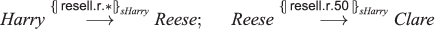to Harry, who, using replacement rule D 3, verifies $\mathit{\text{Harry}}\stackrel{{\left\{\phantom{\rule{0.3em}{0ex}}|\phantom{\rule{0.3em}{0ex}}\mathsf{\text{resell.r.50}}\phantom{\rule{0.3em}{0ex}}|\phantom{\rule{0.3em}{0ex}}\right\}}_{\mathit{\text{sHarry}}}}{\to }\mathit{\text{Clare}}$. Reconsidering the subterfuge attack, even if Mike delegates a copy of the signed permission { | resell.r.* | } sHarry originating from Harry and tricks Reese into thinking he (Mike) is Harry, then when Eve presents the chainto Harry, then, as originator, Harry can not infer $\mathit{\text{Harry}}\stackrel{{\left\{\phantom{\rule{0.3em}{0ex}}|\phantom{\rule{0.3em}{0ex}}\mathsf{\text{resell.r.20}}\phantom{\rule{0.3em}{0ex}}|\phantom{\rule{0.3em}{0ex}}\right\}}_{\mathit{\text{sHarry}}}}{\to }\mathit{\text{Eve}}$.

Given the relative simplicity of the delegation model used in this paper it is not unreasonable to rely on an intuitive argument that subterfuge freedom can be ensured on the basis of its three delegation rules D 1, D 2 and D 3. However, relying solely on an intuitive argument is less convincing for other more complex/expressive delegation models. For example, an intuitive argument for subterfuge freedom is less convincing for a delegation model  that is defined in terms of over twenty delegation rules/axioms. Therefore, we are interested in characterizing subterfuge-freedom as a property so that the rules and operation of a delegation model, such as that used in the reseller example, can be formally verified to be subterfuge-free. In the case of  this analysis would give us confidence that its twenty-plus axioms actually capture what is intended.

## Delegation as a safety property

A delegation system is defined to be a system that carries out operations, on behalf of a principal, based on a collection of delegation certificates that it maintains. The implementation of Reese’s reselling service, along with its use of the delegation rules D 1– D 3, is an example of a delegation system.

Delegation state. Let STATE represent the set of all possible states of a delegation system. For the purposes of this paper we do not consider functional properties of the system and define a state g to be simply the current delegation graph that is accessible by the system. This graph may be stored locally by the system, hosted by an authorization server, distributed among peers, or some combination. Given state g then $P\stackrel{X}{{\to }_{\phantom{\rule{0.3em}{0ex}}\phantom{\rule{0.3em}{0ex}}g}}Q$ denotes delegation of permission X by principal P to principal Q in state g.

Delegation system. The implementation of a delegation system is characterized in terms of a predicate S y s t e m(g), whereby S y s t e m(g) is true iff delegation network g is a reachable state of the implementation.

Delegation policy. Let a delegation policy be a set of delegation states that are considered to be valid. A policy is defined as a predicate P o l i c y(g), whereby P o l i c y(g) is true iff the delegation graph g is considered valid.

For example, the policy for the hotel reseller in Section ‘Example: trust management for service reselling’ is that any room resold by Reese is at least at the minimum-sell rate for the hotel. In particular, if state g indicates that Reese sold a room r in hotel H for rate v then Reese is willing to sell that hotel room at that rate, that is,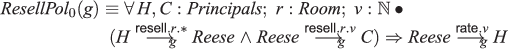The reader should recall the encoding of the minRate relationship as a delegation $\mathit{\text{Reese}}\stackrel{\mathsf{\text{rate}}\mathrm{.u}}{\to }\mathit{\text{Harry}}$, where $\mathsf{\text{rate}}\mathrm{.u}⊑\mathsf{\text{rate}}\mathrm{.v}\phantom{\rule{2.77626pt}{0ex}}⇔\phantom{\rule{2.77626pt}{0ex}}u\ge v$. Given that Reese signed $\mathit{\text{Reese}}\stackrel{\mathsf{\text{rate}}.40}{{\to }_{\phantom{\rule{0.3em}{0ex}}\phantom{\rule{0.3em}{0ex}}g}}\mathit{\text{Harry}}$ and that $\mathsf{\text{rate}}.60⊑\mathsf{\text{rate}}.40$ we can infer by Rule D 2 that $\mathit{\text{Reese}}\stackrel{\mathsf{\text{rate}}.60}{{\to }_{\phantom{\rule{0.3em}{0ex}}\phantom{\rule{0.3em}{0ex}}g}}\mathit{\text{Harry}}$. Thus, the sale $\mathit{\text{Reese}}\stackrel{\mathsf{\text{resell}}\mathrm{.r.}50}{{\to }_{\phantom{\rule{0.3em}{0ex}}\phantom{\rule{0.3em}{0ex}}g}}\mathit{\text{Clare}}$ is valid.

Safe delegation. A delegation system S y s t e m(g) safely upholds a delegation policy P o l i c y(g) every state reachable by the system upholds the delegation policy.

$\forall g:\mathit{\text{STATE}}\bullet \mathit{\text{System}}\left(g\right)⇒\mathit{\text{Policy}}\left(g\right)$
(1)

In constructing S y s t e m(g), Section ‘A poor implementation of the hotel reseller’ considers the delegation model based on the original inference rule D 3 when carrying out certificate reduction in g.

### A poor implementation of the hotel reseller

Suppose that Reese only enters into new contracts from hotels with which she does not already have a contract (identified as having no minimum rate information). As noted previously, for the sake of simplicity, we do not consider management of the contract permission delegations and the creation of a new contract in state g with hotel H corresponds to the setting of a minRate rate v using delegation state transition operation: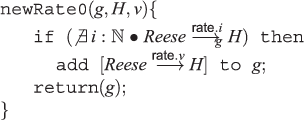Having decided a minimum resell rate for a hotel, Reese engages state transition operation newRoom0newRoom0(g,H,r) whenever she receives a resell delegation statement $H\stackrel{\mathsf{\text{resell}}\mathrm{.r.}\ast }{{}_{\to }}\mathit{\text{Reese}}$ for room r in Hotel H.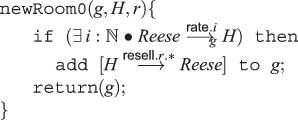Having decided a suitable price v at which to resell hotel H’s room r to customer C, Reese engages state transition operation bookRoom0bookRoom0(g,H,C,r,v) in state g to issue the booking.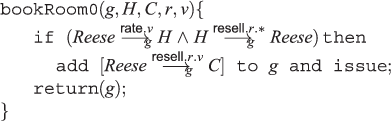Reese’s rationale in this implementation is that, regardless of however she may decide the selling price, then so long as she only uses transitions newRate0newRate0, newRoom0 newRoom0 and bookRoom0 bookRoom0 to update her delegation graph and issue certificates then she will never violate her minimum selling policy.

#### Proposition1.

If we define R e s e l l S y s 0(g) to be the set of all states reachable from an empty graph (initial state) by operations newRate0newRate0, newRoom0 newRoom0 and bookRoom0 bookRoom0 then Reese can prove that every reachable state in her implementation upholds her resell delegation policy, that is,

$\forall g:\mathit{\text{STATE}}\bullet \mathit{\text{ResellSy}}{s}_{0}\left(g\right)⇒\mathit{\text{ResellPo}}{l}_{0}\left(g\right)$
(2)

That is, R e s e l l S y s 0 provides safe delegation under R e s e l l P o l 0. In constructing R e s e l l S y s 0(g) we assume that Reese uses the original inference rule D 3 when carrying out certificate reduction in g.

The proof of Proposition 1 characterizes delegation correctness as a refinement that can be considered to be a safety-style property in the Alpern-Schneider sense . However, the subterfuge example in Section ‘Subterfuge’ demonstrates that such a characterization, as a property on a state, is inadequate (at least to the extent that our characterization of delegation correctness can be considered to represent a safety property). This is not surprising: a frequent argument ,, that is that security properties are not safety properties, but properties over sets of states. This is considered in the next section.

## Delegation as a security property

A principal operating a delegation system may not be certain about the entirety of its delegation state g as a portion of it may have been perturbed by an attacker. The perturbation in the delegation state may come about from an attacker concealing the existence of selected delegation statements and/or injecting new delegation statements. Like a Dolev-Yao attacker , we assume that the attacker can only intercept, copy and paste signed statements, and cannot forge cryptographic signatures.

Delegation state equivalence. Let ≈ be an invariant relation over delegation states whereby g R h is interpreted to mean that principal R is as certain of being in state g as it is of being in state h.

An example of a definition of this equivalence relation is:

$g{\approx }_{R}h\equiv \forall Q:\mathit{\text{Principal}};X:\mathit{\text{PERM}}\bullet R\stackrel{X}{{\to }_{\phantom{\rule{0.3em}{0ex}}\phantom{\rule{0.3em}{0ex}}g}}Q\phantom{\rule{2.77626pt}{0ex}}⇔\phantom{\rule{2.77626pt}{0ex}}R\stackrel{X}{{\to }_{\phantom{\rule{0.3em}{0ex}}\phantom{\rule{0.3em}{0ex}}h}}Q$
(3)

This reflects an assumption that principal R cannot rely on fully knowing the delegations of others and, therefore, the only thing that principal R can be sure about is the delegation statements that it has directly made itself.

Alternatively, suppose that the principle R had a reliable network connection with a set of principles $\mathcal{S}$. This is interpreted to mean that R knows the delegation statements that the principals in $\mathcal{S}$ have or have not made. For example, $\mathcal{S}$ might represent the principals over which a trusted authorization server has jurisdiction and to which R has a reliable connection. Alternatively, for example, the credentials might have been exchanged using a non-repudiation protocol . In these cases, and assuming $R\in \mathcal{S}$, then state equivalence can be generalized to:

$g{\approx }_{R}h\equiv \forall P:\mathcal{S};Q:\mathit{\text{Principal}};X:\mathit{\text{PERM}}\bullet P\stackrel{X}{{\to }_{\phantom{\rule{0.3em}{0ex}}\phantom{\rule{0.3em}{0ex}}g}}Q\phantom{\rule{2.77626pt}{0ex}}⇔\phantom{\rule{2.77626pt}{0ex}}P\stackrel{X}{{\to }_{\phantom{\rule{0.3em}{0ex}}\phantom{\rule{0.3em}{0ex}}h}}Q$

Returning to the hotel reseller example, Reese does not have a reliable connection to any of the hotels and, therefore, cannot be sure about the absence or otherwise of statements she has not directly signed herself. For example, if she does not hold statement $\mathit{\text{Harry}}\stackrel{\mathsf{\text{resell.r.*}}}{{\to }_{\phantom{\rule{0.3em}{0ex}}\phantom{\rule{0.3em}{0ex}}h}}\mathit{\text{Reese}}$ she cannot be sure that it has not been said by Harry and thus we have the state equivalence:Note that Reese can be sure about the minimum selling rate since $\mathit{\text{Reese}}\stackrel{\mathsf{\text{rate}}\mathrm{.v}}{\to }H$ is a delegation statement that she makes directly and stores locally.

A delegation Policy defines a valid delegation state under an assumption that there is certainty about the delegation statements in terms of which it is defined. A principal implementing a delegation System cannot make this assumption and the uncertainty must be considered when deciding whether it is safe to issue a delegation certificate. Therefore, an implementation system in some state g should uphold not just P o l i c y(g) but should also uphold the policy for any other uncertain state that is potentially equivalent.

Secure delegation (subterfuge freedom). A delegation System used by principal R is resilient to subterfuge when upholding a delegation Policy if the delegation policy is upheld by the system for every delegation state h that R can be as certain it is in as each reachable state g.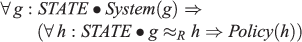### Subterfuge in the original hotel reseller

Consider again the attack on the hotel reseller in Section ‘Subterfuge’. Suppose that Reese has had a series of legitimate interactions with hotels leading to delegation state f, containing a number of sales/bookings and minimum sell rates. Harry then issues a new resell certificate for room rx, which Mike intercepts and conceals and issues a resell certificate of his own for room rx. Reese accepts this resell certificate from Mike and sells the room to Eve:The resulting delegation state of Reese is:

$g=\left[\mathit{\text{Mike}}\stackrel{\mathsf{\text{resell.rx.*}}}{\to }\mathit{\text{Reese}};\phantom{\rule{1em}{0ex}}\phantom{\rule{1em}{0ex}}\phantom{\rule{1em}{0ex}}\mathit{\text{Reese}}\stackrel{\mathsf{\text{resell.rx.20}}}{\to }\mathit{\text{Eve}}\right]\cup \phantom{\rule{2.77626pt}{0ex}}f$

and R e s e l l P o l 0(g) holds. However, Reese is not certain that g represents the complete state, and there is an alternative state h, where g Reese h (based on Equation (3) above), to which the policy should also apply:

$h\phantom{\rule{0.3em}{0ex}}\stackrel{̂}{=}\left[\mathit{\text{Harry}}\stackrel{\mathsf{\text{resell.rx.*}}}{\to }\mathit{\text{Reese}};\phantom{\rule{1em}{0ex}}\phantom{\rule{1em}{0ex}}\phantom{\rule{1em}{0ex}}\mathit{\text{Reese}}\stackrel{\mathsf{\text{resell.rx.20}}}{\to }\mathit{\text{Eve}}\right]\cup \phantom{\rule{2.77626pt}{0ex}}f$

This state h violates the minRate policy. In particular, $\mathit{\text{Reese}}\stackrel{\mathsf{\text{rate.20}}}{{\to }_{\phantom{\rule{0.3em}{0ex}}\phantom{\rule{0.3em}{0ex}}h}}\mathit{\text{Harry}}$ does not hold and therefore R e s e l l P o l 0(h) does not hold. Thus, we have a state g of the system such that

$\mathit{\text{ResellSy}}{s}_{0}\left(g\right)\wedge g{\approx }_{\mathit{\text{Reese}}}h\wedge ¬\phantom{\rule{0.3em}{0ex}}\mathit{\text{ResellPo}}{l}_{0}\left(h\right)$

and, therefore, the system is not subterfuge free.

### Subterfuge-free hotel reseller

Consider the revised reseller delegation mechanism outlined in Section ‘Eliminating subterfuge’. The principals use signed permissions along with the revised reduction rule D 3. We modify the minimum-sell R e s e l l P o l 0 defined in Section ‘Delegation as a safety property’ in order to consider the new permission syntax:As before, the policy is concerned only with enforcing the minimum selling rule, regardless of who may have signed the original permission or how it is implemented. In this way R e s e l l P o l 1 matches the requirements of the original specification of R e s e l l P o l 0 in Section ‘Eliminating subterfuge’. Indeed, a simple translation of R e s e l l S y s 0 to support signed permissions would provide safe delegation under R e s e l l P o l 1 (safety property), while failing to provide secure delegation under the same policy (security property).

The state transition operations are revised to incorporate a new implementation decision that resell permissions must be signed by the delegating hotel. On receipt of a new room resell certificate $H\stackrel{{\left\{\phantom{\rule{0.3em}{0ex}}|\phantom{\rule{0.3em}{0ex}}\mathrm{resell.r.}\ast \phantom{\rule{0.3em}{0ex}}|\phantom{\rule{0.3em}{0ex}}\right\}}_{\mathit{\text{sK}}}}{{}_{\to }}\mathit{\text{Reese}}$ from hotel H for room r (signed by some K) she engages the operation: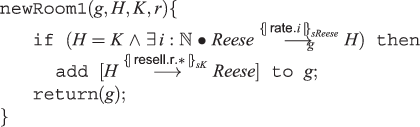Operation bookRoom1 bookRoom1 is similarly revised: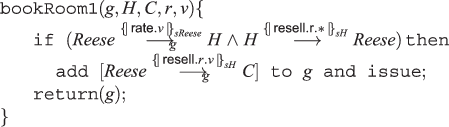and operation newRate1 newRate1 is defined in terms of signed rates: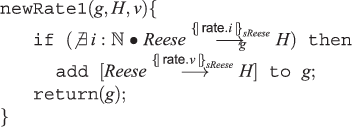These operations along with revised reduction rule D 3 are used to describe the corrected delegation system implementation R e s e l l S y s 1.

The definition of the delegation state equivalence invariant ≈ is unchanged from Equation (3), since whoever may have signed the permission has no impact over what part of the delegation state might be concealed by an attacker.

The sample subterfuge attack no longer works. If Reese is in a state with $\left[\mathit{\text{Mike}}\stackrel{{\left\{\phantom{\rule{0.3em}{0ex}}|\phantom{\rule{0.3em}{0ex}}\mathsf{\text{resell.r.*}}\phantom{\rule{0.3em}{0ex}}|\phantom{\rule{0.3em}{0ex}}\right\}}_{\mathit{\text{sHarry}}}}{{\to }_{\phantom{\rule{0.3em}{0ex}}\phantom{\rule{0.3em}{0ex}}g}}\mathit{\text{Reese}}\right]$ then the new bookRoom bookRoom operation will not delegate (on behalf of Reese) permission { | resell.r.20 | } sHarry to Eve since the delegator Mike of the permission held by Reese is not the signer Harry of the permission.

#### Proposition2.

R e s e l l S y s 1 provides secure delegation under R e s e l l P o l 1:

$\forall g,h:\mathit{\text{STATE}}\bullet \left(\mathit{\text{ResellSy}}{s}_{1}\left(g\right)\wedge g{\approx }_{\mathit{\text{Reese}}}h\right)⇒\mathit{\text{ResellPo}}{l}_{1}\left(h\right)$

#### Proof.

Let s p e a k e r s(g) be the set of principals who have signed/made delegation statements in g. We prove by induction that

$\forall g:\mathit{\text{STATE}}\bullet \mathit{\text{ResellSy}}{s}_{1}\left(g\right)⇒\left(\forall h:\mathit{\text{STATE}}\bullet g{\approx }_{R}h⇒\mathit{\text{ResellPo}}{l}_{1}\left(h\right)\right)$

Base case. Given an initial delegation state i n i t= [ ] then it follows that R e s e l l S y s 1(i n i t). Consider any equivalent state h where i n i t R h, then by definition we have Rs p e a k e r s(h). Thus, there is no delegation of the form $R\stackrel{{\left\{\phantom{\rule{0.3em}{0ex}}|\phantom{\rule{0.3em}{0ex}}\mathrm{resell.r.v}\phantom{\rule{0.3em}{0ex}}|\phantom{\rule{0.3em}{0ex}}\right\}}_{\mathit{\text{sK}}}}{{\to }_{\phantom{\rule{0.3em}{0ex}}\phantom{\rule{0.3em}{0ex}}h}}C$ in state h and, therefore, R e s e l l P o l 1(h) holds. Therefore, the base case holds.

Inductive step. Assume that R e s e l l S y s 1 is in a state g where (h:S T A T Eg R hR e s e l l P o l 1(h)). The inductive goal is to prove that for all h :S T A T E then o p(g)≈ R h R e s e l l P o l 1(h )), for each system transition operation op.

Consider each system transition g =o p(g). If the operation precondition is satisfied then g=g and the inductive goal trivially holds. Consider g when the operation precondition holds.

g =newRate1(g,H,v): given precondition $\left(\nexists i:ℕ\bullet R\stackrel{{\left\{\phantom{\rule{0.3em}{0ex}}|\phantom{\rule{0.3em}{0ex}}\mathrm{rate.i}\phantom{\rule{0.3em}{0ex}}|\phantom{\rule{0.3em}{0ex}}\right\}}_{R}}{{\to }_{\phantom{\rule{0.3em}{0ex}}\phantom{\rule{0.3em}{0ex}}g}}H\right)$ then ${g}^{\prime }=g\cup \left[\phantom{\rule{0.3em}{0ex}}R\stackrel{{\left\{\phantom{\rule{0.3em}{0ex}}|\phantom{\rule{0.3em}{0ex}}\mathrm{rate.v}\phantom{\rule{0.3em}{0ex}}|\phantom{\rule{0.3em}{0ex}}\right\}}_{R}}{{}_{\to }}H\right]$. Consider a state h where g R h . It follows from the definition of equivalence that $\left[\phantom{\rule{0.3em}{0ex}}R\stackrel{{\left\{\phantom{\rule{0.3em}{0ex}}|\phantom{\rule{0.3em}{0ex}}\mathrm{rate.v}\phantom{\rule{0.3em}{0ex}}|\phantom{\rule{0.3em}{0ex}}\right\}}_{R}}{{}_{\to }}H\right]\in {h}^{\prime }$, and thus if $h={h}^{\prime }/\phantom{\rule{0.3em}{0ex}}\left[\phantom{\rule{0.3em}{0ex}}R\stackrel{{\left\{\phantom{\rule{0.3em}{0ex}}|\phantom{\rule{0.3em}{0ex}}\mathrm{rate.v}\phantom{\rule{0.3em}{0ex}}|\phantom{\rule{0.3em}{0ex}}\right\}}_{R}}{{}_{\to }}H\right]$ then g R h. Therefore, given the inductive hypothesis, R e s e l l P o l 1(h) holds. Suppose $\mathit{\text{ResellPo}}{l}_{1}\phantom{\rule{0.3em}{0ex}}\left(h\cup \phantom{\rule{0.3em}{0ex}}\left[\phantom{\rule{0.3em}{0ex}}R\stackrel{{\left\{\phantom{\rule{0.3em}{0ex}}|\phantom{\rule{0.3em}{0ex}}\mathrm{rate.v}\phantom{\rule{0.3em}{0ex}}|\phantom{\rule{0.3em}{0ex}}\right\}}_{R}}{{}_{\to }}H\right]\right)$ is false; for this to be the case, the R e s e l l P o l 1(h ) antecedent $\left(H\stackrel{{\left\{\phantom{\rule{0.3em}{0ex}}|\phantom{\rule{0.3em}{0ex}}\mathrm{resell.r.}\ast \phantom{\rule{0.3em}{0ex}}|\phantom{\rule{0.3em}{0ex}}\right\}}_{\mathit{\text{sK}}}}{{\to }_{\phantom{\rule{0.3em}{0ex}}\phantom{\rule{0.3em}{0ex}}{h}^{\prime }}}R\wedge R\stackrel{{\left\{\phantom{\rule{0.3em}{0ex}}|\phantom{\rule{0.3em}{0ex}}\mathrm{resell.r.u}\phantom{\rule{0.3em}{0ex}}|\phantom{\rule{0.3em}{0ex}}\right\}}_{\mathit{\text{sK}}}}{{\to }_{\phantom{\rule{0.3em}{0ex}}\phantom{\rule{0.3em}{0ex}}{h}^{\prime }}}C\right)$ has a negative conclusion $\left[\phantom{\rule{0.3em}{0ex}}¬R\stackrel{{\left\{\phantom{\rule{0.3em}{0ex}}|\phantom{\rule{0.3em}{0ex}}\mathrm{rate.u}\phantom{\rule{0.3em}{0ex}}|\phantom{\rule{0.3em}{0ex}}\right\}}_{R}}{{\to }_{\phantom{\rule{0.3em}{0ex}}\phantom{\rule{0.3em}{0ex}}{h}^{\prime }}}H\right]$. However, if R h { | r e s e l l.r.u | } sK C holds in state h then, by g R h, $R\stackrel{{\left\{\phantom{\rule{0.3em}{0ex}}|\phantom{\rule{0.3em}{0ex}}\mathrm{resell.r.u}\phantom{\rule{0.3em}{0ex}}|\phantom{\rule{0.3em}{0ex}}\right\}}_{\mathit{\text{sK}}}}{{\to }_{\phantom{\rule{0.3em}{0ex}}\phantom{\rule{0.3em}{0ex}}g}}C$ must also hold in state g. However, the system cannot be in a state g where a resell certificate is issued without a corresponding rate statement. Therefore, this antecedent is false and thus, given g R h then R e s e l l P o l 1(h ) holds.

g =newRoom1(g,H,K,r). For precondition $\left(\exists i:ℕ\bullet R\stackrel{{\left\{\phantom{\rule{0.3em}{0ex}}|\phantom{\rule{0.3em}{0ex}}\mathrm{rate.i}\phantom{\rule{0.3em}{0ex}}|\phantom{\rule{0.3em}{0ex}}\right\}}_{\mathit{\text{sR}}}}{{\to }_{\phantom{\rule{0.3em}{0ex}}\phantom{\rule{0.3em}{0ex}}g}}H\right)$ then ${g}^{\prime }=g\cup \phantom{\rule{0.3em}{0ex}}\left[\phantom{\rule{0.3em}{0ex}}H\stackrel{{\left\{\phantom{\rule{0.3em}{0ex}}|\phantom{\rule{0.3em}{0ex}}\mathrm{resell.r.}\ast \phantom{\rule{0.3em}{0ex}}|\phantom{\rule{0.3em}{0ex}}\right\}}_{\mathit{\text{sH}}}}{{}_{\to }}R\right]$. By the definition of equivalence, we have g R g , and therefore, by transitivity of equivalence, for any state h such that g R h holds, then the inductive hypothesis gives g R h R e s e l l P o l 1(h ).

g =bookRoom1(g,H,C,r,v). Given precondition $\left(\phantom{\rule{0.3em}{0ex}}R\phantom{\rule{0.3em}{0ex}}\stackrel{{\left\{\phantom{\rule{0.3em}{0ex}}|\phantom{\rule{0.3em}{0ex}}\mathrm{rate.v}\phantom{\rule{0.3em}{0ex}}|\phantom{\rule{0.3em}{0ex}}\right\}}_{\mathit{\text{sR}}}}{{\to }_{\phantom{\rule{0.3em}{0ex}}\phantom{\rule{0.3em}{0ex}}g}}H\wedge H\stackrel{{\left\{\phantom{\rule{0.3em}{0ex}}|\phantom{\rule{0.3em}{0ex}}\mathrm{resell.r.}\ast \phantom{\rule{0.3em}{0ex}}|\phantom{\rule{0.3em}{0ex}}\right\}}_{\mathit{\text{sH}}}}{{}_{\to }}\phantom{\rule{0.3em}{0ex}}R\phantom{\rule{0.3em}{0ex}}\right)$ then ${g}^{\prime }=g\cup \phantom{\rule{0.3em}{0ex}}\left[R\stackrel{{\left\{\phantom{\rule{0.3em}{0ex}}|\phantom{\rule{0.3em}{0ex}}\mathrm{resell.r.v}\phantom{\rule{0.3em}{0ex}}|\phantom{\rule{0.3em}{0ex}}\right\}}_{\mathit{\text{sH}}}}{{\to }_{\phantom{\rule{0.3em}{0ex}}\phantom{\rule{0.3em}{0ex}}g}}C\right]$. Consider a state h where g R h . It follows from the definition of equivalence that $\left[R\stackrel{{\left\{\phantom{\rule{0.3em}{0ex}}|\phantom{\rule{0.3em}{0ex}}\mathrm{resell.r.v}\phantom{\rule{0.3em}{0ex}}|\phantom{\rule{0.3em}{0ex}}\right\}}_{\mathit{\text{sH}}}}{{\to }_{\phantom{\rule{0.3em}{0ex}}\phantom{\rule{0.3em}{0ex}}g}}C\right]\in {h}^{\prime }$, and thus if $h={h}^{\prime }/\phantom{\rule{0.3em}{0ex}}\left[\phantom{\rule{0.3em}{0ex}}R\stackrel{{\left\{\phantom{\rule{0.3em}{0ex}}|\phantom{\rule{0.3em}{0ex}}\mathrm{resell.r.v}\phantom{\rule{0.3em}{0ex}}|\phantom{\rule{0.3em}{0ex}}\right\}}_{\mathit{\text{sH}}}}{{\to }_{\phantom{\rule{0.3em}{0ex}}\phantom{\rule{0.3em}{0ex}}g}}C\right]$ then g R h. Therefore, given the inductive hypothesis, R e s e l l P o l 1(h) holds. Given g R h then a rate statement in g appears in h and if the precondition of newRoom1 newRoom1 holds in g then it also holds in h . Therefore, if the resell action is valid in g it will also be valid in h and R e s e l l P o l 1(h ) holds. □

The purpose of this paper is to provide a formal definition for subterfuge freedom. The example above illustrates that defining it as a safety-style property on delegation states is inadequate. The proposed definition of subterfuge freedom is defined as a property on sets of delegation states, which is not surprising, given that security properties are generally characterized as a properties on sets of states.

The R e s e l l S y s 1 system is an example of a system that uses the specific Rules D 1, D 2 and D 3 to deduce whether it is secure to carry out some requested action, such as accept a room rate from a customer. Rather than simply accept an intuitive argument that using these rules ensure subterfuge freedom, Proposition 2 formally proves that it is not possible for an attacker to carry out a subterfuge attack on this system. The definition of subterfuge-freedom is not limited to systems built using only rules D 1, D 2 and D 3; defining subterfuge-freedom as a property means that it can be used to verify the subterfuge freedom of systems designed to use different rules to reason over delegation statements.

Our formalization of subterfuge freedom is limited to delegation systems defined over delegation statements of the form $P\stackrel{X}{\to }Q$. Therefore, in principle, it could be used to validate systems that use revised (subterfuge-safe) rules for delegation schemes such as SPKI/SDSI, KeyNote and X509 attribute certificates. We are currently using the property to analyze the subterfuge freedom of the delegation model in , which is defined in terms of twenty-plus deduction rules.

## Related work

Trust Management systems such as –, are intended to provide a decentralized approach to constructing and interpreting authorization relationships between principals/domains. Unlike a centralized authorization server-based approach, authorization rules are defined and signed locally by issuing principals. These cryptographic delegation credentials can be distributed in any manner to suit the design of the (Trust Management-based) access control mechanism that mediates according to the policy. In this way, trust management provides a basis for secure decentralized policy based management.

While credential-based policy rules are inherently decentralized, many implicitly assume unique and unambiguous global permissions, effectively originating from some central authority that provides a permission namespace that everyone agrees to consistently use. For example, Keynote  suggests using the Internet Assigned Number Authority (IANA), RT  relies on Application Domain Specification Documents (ADSDs), and X509 relies on the X500 name service, to ensure that different parties use the right name for resources, conditions, other participants, and so forth. However, principals may prefer not to have to trust some global authority, irrespective of the practicalities of such an authority.

Delegation subterfuge , arises when there is ambiguity in interpreting a permission in its namespace. This can come about from an attacker concealing and/or injecting delegation credentials whereby, as a consequence, a victim may violate the policy that guides its own delegation actions, as illustrated in this paper. Under reasonable assumptions, public keys can be considered to be globally unique and, by signing a permission, a principal can be sure that the resulting value is globally unique. Subterfuge-freedom can be provided in a role-based distributed authorization language by constraining delegation to permissions that have an associated originating public key . While effective, this approach suffers the challenge of reliably referencing public keys. A SDSI-like naming system can alternatively be used to provide subterfuge-free local permission namespaces . The FRM distributed policy management framework  also relies on signed permissions to avoid subterfuge and uses Distinguished Names/X509 certificates to uniquely tie a permission to its namespace. While seeking to avoid subterfuge-like vulnerabilities, these schemes are justified on the basis of intuitive argument rather than on a formal definition of the a meaning of subterfuge.

While subterfuge is concerned with how an attacker can interfere with a target’s policy certificates, probing-free authorization,, is concerned with determining what an attacker can infer about hidden policy certificates when making queries. Both subterfuge and probing are effectively concerned with determining whether information flows ,,, from one principal to another as a result of using the delegation system. Further investigating the relationships between subterfuge and probing, and their relationship to information flow properties in delegation systems is an open topic of research. One avenue of interest is whether intransitive information flow ,, might provide an interpretation for conditional subterfuge-freedom. In this case, a principal may declare that it is willing to accept accountability for some third-party’s permission with the consequence that any perturbation to its delegation state prior to the declaration can be safely ignored.

## Discussion and conclusion

Security refinement can be defined to be a system robustly upholding functional requirements (policy) in the presence of threats that may perturb a state that has been arrived at via some trace . If one considers delegation states to be analogous to system traces then the definition of delegation security/subterfuge freedom proposed in this paper is similar, at least in intent, to this definition.

We argue that subterfuge-freedom is not a safety-style property in the conventional sense , but that it is a security property ,, that is similar to non-interference ,,,. This paper presents an example of an implementation of a policy requirement that appears to be preserved under a safety refinement (Proposition 1), but which is subject to a subterfuge attack. Section ‘Delegation as a security property’ demonstrates that the implementation of the policy requirement is not preserved under the proposed security-style refinement. By using a restricted form of (subterfuge-free) delegation, a revised implementation can be shown to preserve the policy under security refinement.

The primary contribution of this paper is a characterization of subterfuge-freedom as a security-style property based on delegation statements of the form $P\stackrel{X}{\to }Q$. To our knowledge, this is the first characterization of subterfuge as a security property. While relatively straightforward, the delegation model provided a sufficient scheme in which to present the result. We hope that this will provide insight into the refinement of the definitions for more expressive authorization logics that support reasoning over richer statements, such as ,. We are currently exploring a Kripke-based semantics with which to more formally investigate subterfuge properties and their relationship to safety and security properties in general.

## References

1. Lampson B, Abadi M, Burrows M, Wobber E: Authentication in distributed systems: theory and pratice. ACM Trans Comput Syst 1992, 10(4):265–310. 10.1145/138873.138874

2. Blaze M, Feigenbaum J, Strauss M: Compliance checking in the policymaker trust management system. In C ‘98: proceedings of the second international conference on financial cryptography. Lecture Notes in Computer Science. Springer, Berlin; 1998:254–274.

3. Li N, Mitchell JC: RT: a role-based trust-management framework. In The Third DARPA information survivability conference and exposition (DISCEX III). IEEE Computer Society Press, Washington, DC; 2003:201–212.

4. Becker MY, Fournet C, Gordon AD: SecPAL: design and semantics of a decentralized authorization language. J Comput Secur 2010, 18(4):619–665.

5. Foley SN, Zhou H: Authorisation subterfuge by delegation in decentralised networks. In International security protocols workshop. Lecture Notes in Computer Science. Springer, Berlin; 2005:97–102.

6. Zhou H, Foley SN: A framework for establishing decentralized secure coalitions. In Proceedings of IEEE computer security foundations workshop. IEEE Computer Society Press, Washington, DC; 2006:270–282.

7. Zhou H, Foley SN: A logic for analysing subterfuge in delegation chains. In Workshop on Formal Aspects in Security and Trust (FAST2005). Lecture Notes in Computer Science. Springer, Berlin; 2005:127–141.

8. Foley SN, Abdi S: Avoiding delegation subterfuge using linked local permission names. In Proceedings of 8th international workshop on Formal Aspects of Security and Trust (FAST2011). Lecture Notes in Computer Science. Springer, Berlin; 2011.

9. Alpern B, Schneider FB: Recognizing safety and liveness. Distr Comput 1987, 2: 117–126. 10.1007/BF01782772

10. Goguen JA: Meseguer J (1984) Unwinding and inference control. In IEEE symposium on security and privacy. IEEE Computer Society Press, Washington, DC; :75–87.

11. Foley SN: A non-functional approach to system integrity. IEEE J Sel Area Comm 2003, 21(1):36–43. 10.1109/JSAC.2002.806124

12. Ellison C, Frantz B, Lampson B, Rivest R, Thomas B, Ylonen T (1999) SPKI Certificate Theory (RFC 2693). Internet Engineering Task Force. http://www.ietf.org/rfc/rfc2693.txt

13. Feeney K, Lewis D, O’Sullivan D: Service oriented policy management for web-application frameworks. IEEE Internet Comput Mag 2009, 13(6):39–47. 10.1109/MIC.2009.95

14. Blaze M, Feigenbaum J, Ioannidis J, Keromytis A (1999) The KeyNote Trust-Management System Version 2 (RFC 2704). Internet Engineering Task Force. http://www.ietf.org/rfc/rfc2704.txt

15. Schneier B: Semantic network attacks. Commun ACM 2000, 43(12):168. 10.1145/355112.355131

16. Blaze M, Ioannidis J, Keromytis AD: Offline micropayments without trusted hardware. In Financial cryptography. Lecture Notes in Computer Science. Springer, Berlin; 2001:21–40.

17. Foley SN: Using trust management to support transferable hash-based micropayments. In Proceedings of the 7th international financial cryptography conference. Lecture Notes in Computer Science. Springer, Berlin; 2003.

18. Blaze M, Ioannidis J, Ioannidis S, Keromytis A, Nikander P, Prevelakis V: Tapi: Transactions for Accessing Public Infrastructure. Springer, Berlin; 2003.

19. Zeller T (2005) Purloined domain name is an unsolved mystery In: New York Times, January 18. http://www.nytimes.com/2005/01/18/technology/18domain.html?_r=0

20. Geer D: Power. law. IEEE Security & Privacy. IEEE Comput Soc 2012, 10(1):94–95.

21. Guidelines for the issuance and management of extended validation certificates. Technical Report Version 1.1.6, Certification Authority/Browser Forum. (2013). https://cabforum.org/wp-content/uploads/Baseline_Requirements_V1_1_6.pdf

22. Ellison CM: The nature of a usable PKI. Comput Networks 1999, 31: 823–830. 10.1016/S1389-1286(98)00018-8

23. Jacob JL: Basic theorems about security. J Comput Security 1992, 1: 385–411.

24. Ryan P: Mathematical models of computer security. In Foundations of security analysis and design. Lecture Notes in Computer Science. Edited by: Focardi R, Gorrieri R. Springer, Berlin; 2001:1–62. 10.1007/3-540-45608-2_1

25. Dolev D, Yao AC: On the security of public key protocols. IEEE Trans Inform Theor 1983, 29(2):198–208. 10.1109/TIT.1983.1056650

26. Zhou J, Gollmann D: An efficient non-repudiation protocol. In 10th computer security foundations workshop. IEEE Computer Society Press, Washington, DC; 1997:126–132.

27. Gurevich Y, Neeman I: DKAL: Distributed-Knowledge Authorization Language. In Proceedings of computer security foundations. IEEE Computer Society, Washington, DC; 2008.

28. Becker MY: Information flow in trust management systems. J Comput Secur 2012, 20(6):677–708.

29. Mantel H: Information flow and noninterference. In Encyclopedia of cryptography and security. Springer, Berlin; 2011:605–607.

30. Foley SN: Aggregation and separation as noninterference properties. J Comput Secur 1992, 1(2):159–188.

31. Roscoe AW, Goldsmith MH: What is intransitive noninterference? In Proceedings of computer security foundations workshop. IEEE Computer Society Press, Washington, DC; 1999:228–238.

32. Engelhardt K, van der Meyden R, Zhang C (2012) Intransitive noninterference in nondeterministic systems In: ACM conference on computer and communications security, 869–880.

## Acknowledgement

This research has been supported in part by Science Foundation Ireland grants SFI 08/SRC/11403 and SFI 10/CE/I1853. The author would like to thank the anonymous reviewers for their helpful feedback.

## Author information

Authors

### Corresponding author

Correspondence to Simon N Foley.

### Competing interests

The author declares that he has no competing interests.

## Authors’ original submitted files for images

Below are the links to the authors’ original submitted files for images.

## Rights and permissions